## The Normal Approximation to the Binomial Distribution

The process of using the normal curve to estimate the shape of the binomial distribution is known as normal approximation.

### Learning Objectives

Explain the origins of central limit theorem for binomial distributions

### Key Takeaways

#### Key Points

• Originally, to solve a problem such as the chance of obtaining 60 heads in 100 coin flips, one had to compute the probability of 60 heads, then the probability of 61 heads, 62 heads, etc, and add up all these probabilities.
• Abraham de Moivre noted that when the number of events (coin flips) increased, the shape of the binomial distribution approached a very smooth curve.
• Therefore, de Moivre reasoned that if he could find a mathematical expression for this curve, he would be able to solve problems such as finding the probability of 60 or more heads out of 100 coin flips much more easily.
• This is exactly what he did, and the curve he discovered is now called the normal curve.

#### Key Terms

• normal approximation: The process of using the normal curve to estimate the shape of the distribution of a data set.
• central limit theorem: The theorem that states: If the sum of independent identically distributed random variables has a finite variance, then it will be (approximately) normally distributed.

The binomial distribution can be used to solve problems such as, “If a fair coin is flipped 100 times, what is the probability of getting 60 or more heads?” The probability of exactly $\text{x}$ heads out of $\text{N}$ flips is computed using the formula:

$\displaystyle \text{P}\left( \text{x} \right) =\frac { \text{N}! }{ \text{x}!\left( \text{N}-\text{x} \right) ! } { \pi }^{ \text{x} }{ \left( 1-\pi \right) }^{ \text{N}-\text{x} }$

where $\text{x}$ is the number of heads (60), $\text{N}$ is the number of flips (100), and $\pi$ is the probability of a head (0.5). Therefore, to solve this problem, you compute the probability of 60 heads, then the probability of 61 heads, 62 heads, etc, and add up all these probabilities.

Abraham de Moivre, an 18th century statistician and consultant to gamblers, was often called upon to make these lengthy computations. de Moivre noted that when the number of events (coin flips) increased, the shape of the binomial distribution approached a very smooth curve. Therefore, de Moivre reasoned that if he could find a mathematical expression for this curve, he would be able to solve problems such as finding the probability of 60 or more heads out of 100 coin flips much more easily. This is exactly what he did, and the curve he discovered is now called the normal curve. The process of using this curve to estimate the shape of the binomial distribution is known as normal approximation.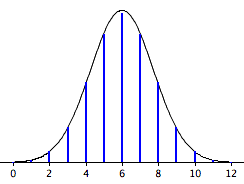Normal Approximation: The normal approximation to the binomial distribution for 12 coin flips. The smooth curve is the normal distribution. Note how well it approximates the binomial probabilities represented by the heights of the blue lines.

The importance of the normal curve stems primarily from the fact that the distribution of many natural phenomena are at least approximately normally distributed. One of the first applications of the normal distribution was to the analysis of errors of measurement made in astronomical observations, errors that occurred because of imperfect instruments and imperfect observers. Galileo in the 17th century noted that these errors were symmetric and that small errors occurred more frequently than large errors. This led to several hypothesized distributions of errors, but it was not until the early 19th century that it was discovered that these errors followed a normal distribution. Independently the mathematicians Adrian (in 1808) and Gauss (in 1809) developed the formula for the normal distribution and showed that errors were fit well by this distribution.

This same distribution had been discovered by Laplace in 1778—when he derived the extremely important central limit theorem. Laplace showed that even if a distribution is not normally distributed, the means of repeated samples from the distribution would be very nearly normal, and that the the larger the sample size, the closer the distribution would be to a normal distribution. Most statistical procedures for testing differences between means assume normal distributions. Because the distribution of means is very close to normal, these tests work well even if the distribution itself is only roughly normal.

## The Scope of the Normal Approximation

The scope of the normal approximation is dependent upon our sample size, becoming more accurate as the sample size grows.

### Learning Objectives

Explain how central limit theorem is applied in normal approximation

### Key Takeaways

#### Key Points

• The tool of normal approximation allows us to approximate the probabilities of random variables for which we don’t know all of the values, or for a very large range of potential values that would be very difficult and time consuming to calculate.
• The scope of the normal approximation follows with the statistical themes of the law of large numbers and central limit theorem.
• According to the law of large numbers, the average of the results obtained from a large number of trials should be close to the expected value, and will tend to become closer as more trials are performed.
• The central limit theorem (CLT) states that, given certain conditions, the mean of a sufficiently large number of independent random variables, each with a well-defined mean and well-defined variance, will be approximately normally distributed.

#### Key Terms

• central limit theorem: The theorem that states: If the sum of independent identically distributed random variables has a finite variance, then it will be (approximately) normally distributed.
• law of large numbers: The statistical tendency toward a fixed ratio in the results when an experiment is repeated a large number of times.
• normal approximation: The process of using the normal curve to estimate the shape of the distribution of a data set.

The tool of normal approximation allows us to approximate the probabilities of random variables for which we don’t know all of the values, or for a very large range of potential values that would be very difficult and time consuming to calculate. We do this by converting the range of values into standardized units and finding the area under the normal curve. A problem arises when there are a limited number of samples, or draws in the case of data “drawn from a box.” A probability histogram of such a set may not resemble the normal curve, and therefore the normal curve will not accurately represent the expected values of the random variables. In other words, the scope of the normal approximation is dependent upon our sample size, becoming more accurate as the sample size grows. This characteristic follows with the statistical themes of the law of large numbers and central limit theorem (reviewed below).Law of Large Numbers: An illustration of the law of large numbers using a particular run of rolls of a single die. As the number of rolls in this run increases, the average of the values of all the results approaches 3.5. While different runs would show a different shape over a small number of throws (at the left), over a large number of rolls (to the right) they would be extremely similar.

### Law of Large Numbers

The law of large numbers (LLN) is a theorem that describes the result of performing the same experiment a large number of times. According to the law, the average of the results obtained from a large number of trials should be close to the expected value, and will tend to become closer as more trials are performed.

The law of large numbers is important because it “guarantees” stable long-term results for the averages of random events. For example, while a casino may lose money in a single spin of the roulette wheel, its earnings will tend towards a predictable percentage over a large number of spins. Any winning streak by a player will eventually be overcome by the parameters of the game. It is important to remember that the LLN only applies (as the name indicates) when a large number of observations are considered. There is no principle that a small number of observations will coincide with the expected value or that a streak of one value will immediately be “balanced” by the others.

### Central Limit Theorem

The central limit theorem (CLT) states that, given certain conditions, the mean of a sufficiently large number of independent random variables, each with a well-defined mean and well-defined variance, will be approximately normally distributed. The central limit theorem has a number of variants. In its common form, the random variables must be identically distributed. In variants, convergence of the mean to the normal distribution also occurs for non-identical distributions, given that they comply with certain conditions.

More precisely, the central limit theorem states that as $\text{n}$ gets larger, the distribution of the difference between the sample average $\text{S}_\text{n}$ and its limit $\mu$, when multiplied by the factor:

$\sqrt { \text{n} }$ (that is $\sqrt { \text{n} } ({ \text{S} }_{ \text{n} }-\mu )$)

Approximates the normal distribution with mean 0 and variance $\sigma^2$. For large enough $\text{n}$, the distribution of $\text{S}_\text{n}$ is close to the normal distribution with mean $\mu$ and variance $\frac { { \sigma }^{ 2 } }{ \text{n} }$. The usefulness of the theorem is that the distribution of $\sqrt { \text{n} } ({ \text{S} }_{ \text{n} }-\mu )$ approaches normality regardless of the shape of the distribution of the individual $\text{X}_\text{i}$‘s.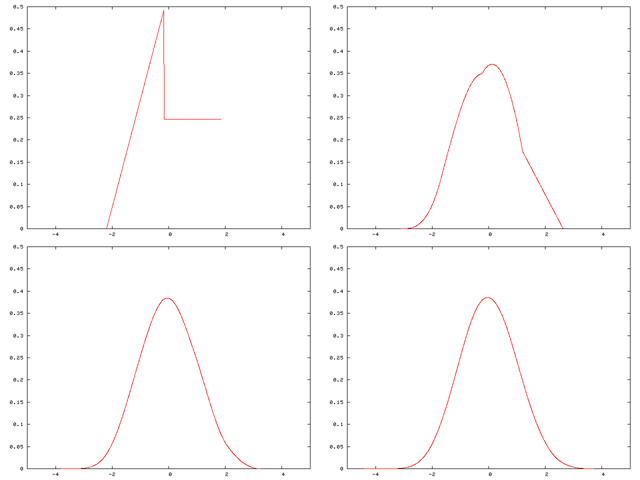Central Limit Theorem: A distribution being “smoothed out” by summation, showing original density of distribution and three subsequent summations

## Calculating a Normal Approximation

In this atom, we provide an example on how to compute a normal approximation for a binomial distribution.

### Learning Objectives

Demonstrate how to compute normal approximation for a binomial distribution

### Key Takeaways

#### Key Points

• In our example, we have a fair coin and wish to know the probability that you would get 8 heads out of 10 flips.
• The binomial distribution has a mean of $\mu = \text{Np} = 10\cdot 0.5 = 5$ and a variance of $\sigma^2 = \text{Np}(1-\text{p}) = 10 \cdot 0.5\cdot 0.5 = 2.5$; therefore a standard deviation of 1.5811.
• A total of 8 heads is 1.8973 standard deviations above the mean of the distribution.
• Because the binomial distribution is discrete an the normal distribution is continuous, we round off and consider any value from 7.5 to 8.5 to represent an outcome of 8 heads.
• Using this approach, we calculate the area under a normal curve (which will be the binomial probability) from 7.5 to 8.5 to be 0.044.

#### Key Terms

• z-score: The standardized value of observation $\text{x}$ from a distribution that has mean $\mu$ and standard deviation $\sigma$.
• binomial distribution: the discrete probability distribution of the number of successes in a sequence of $n$ independent yes/no experiments, each of which yields success with probability $p$

The following is an example on how to compute a normal approximation for a binomial distribution.

Assume you have a fair coin and wish to know the probability that you would get 8 heads out of 10 flips. The binomial distribution has a mean of $\mu = \text{Np} = 10\cdot 0.5 = 5$ and a variance of $\sigma^2 = \text{Np}(1-\text{p}) = 10 \cdot 0.5\cdot 0.5 = 2.5$. The standard deviation is, therefore, 1.5811. A total of 8 heads is:

$\displaystyle \frac { 8-5 }{ 1.5811 } =1.8973$

Standard deviations above the mean of the distribution. The question then is, “What is the probability of getting a value exactly 1.8973 standard deviations above the mean?” You may be surprised to learn that the answer is 0 (the probability of any one specific point is 0). The problem is that the binomial distribution is a discrete probablility distribution whereas the normal distribultion is a continuous distribution.

The solution is to round off and consider any value from 7.5 to 8.5 to represent an outcome of 8 heads. Using this approach, we calculate the area under a normal curve from 7.5 to 8.5. The area in green in the figure is an approximation of the probability of obtaining 8 heads.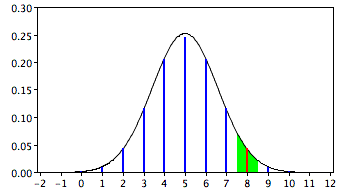Normal Approximation: Approximation for the probability of 8 heads with the normal distribution.

To calculate this area, first we compute the area below 8.5 and then subtract the area below 7.5. This can be done by finding $\text{z}$-scores and using the $\text{z}$-score table. Here, for the sake of ease, we have used an online normal area calculator. The results are shown in the following figures: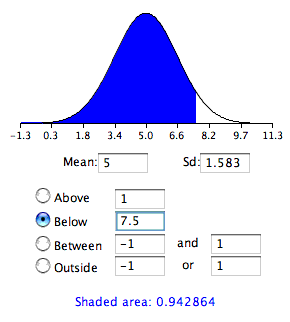Normal Area 2: This graph shows the area below 7.5.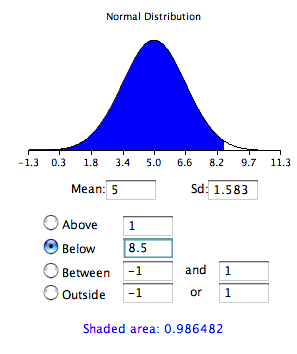Normal Area 1: This graph shows the area below 8.5.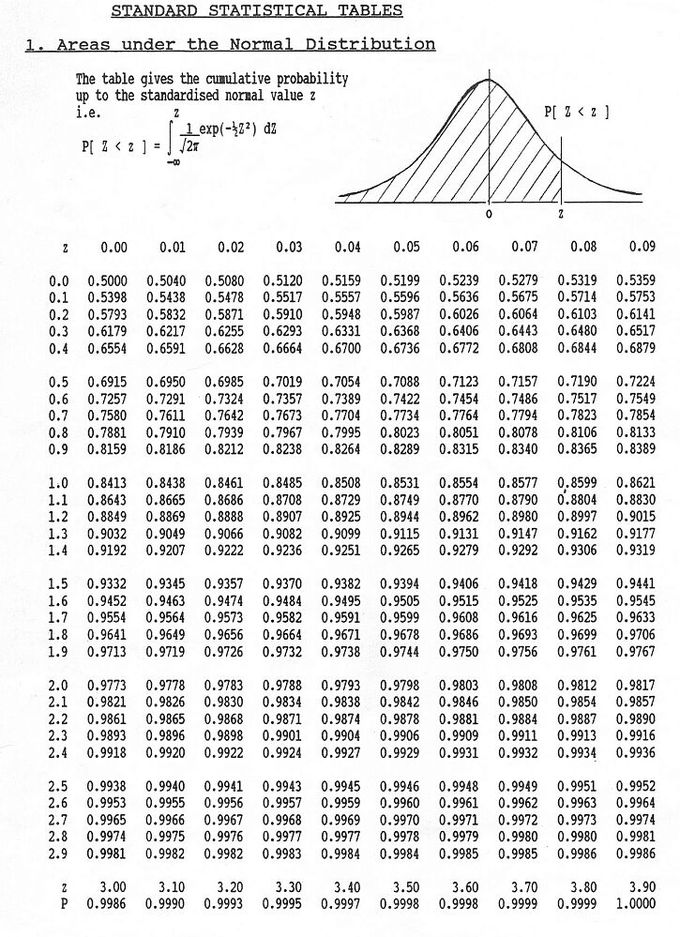$\text{z}$-Score Table: The $\text{z}$-score table is used to calculate probabilities for the standard normal distribution.

The differences between the areas is 0.044, which is the approximation of the binomial probability. For these parameters, the approximation is very accurate. If we did not have the normal area calculator, we could find the solution using a table of the standard normal distribution (a $\text{z}$-table) as follows:

1. Find a $\text{Z}$ score for 7.5 using the formula $\text{Z}=\frac { 7.5-5 }{ 1.5811 } =1.5811$
2. Find the area below a $\text{Z}$ of $1.58=0.943$.
3. Find a $\text{Z}$ score for 8.5 using the formula $\text{Z}=\frac { 8.5-5 }{ 1.5811 } =2.21$
4. Find the area below a $\text{Z}$ of $2.21=0.987$.
5. Subtract the value in step 2 from the value in step 4 to get 0.044.

The same logic applies when calculating the probability of a range of outcomes. For example, to calculate the probability of 8 to 10 flips, calculate the area from 7.5 to 10.5.

## Change of Scale

In order to consider a normal distribution or normal approximation, a standard scale or standard units is necessary.

### Learning Objectives

Explain the significance of normalization of ratings and calculate this normalization

### Key Takeaways

#### Key Points

• In the simplest cases, normalization of ratings means adjusting values measured on different scales to a notionally common scale, often prior to averaging.
• In more complicated cases, normalization may refer to more sophisticated adjustments where the intention is to bring the entire probability distributions of adjusted values into alignment.
• The standard score is a dimensionless quantity obtained by subtracting the population mean from an individual raw score and then dividing the difference by the population standard deviation.
• A key point is that calculating $\text{z}$ requires the population mean and the population standard deviation, not the sample mean or sample deviation.

#### Key Terms

• datum: A measurement of something on a scale understood by both the recorder (a person or device) and the reader (another person or device).
• standard score: The number of standard deviations an observation or datum is above the mean.
• normalization: The process of removing statistical error in repeated measured data.

In order to consider a normal distribution or normal approximation, a standard scale or standard units is necessary.

### Normalization

In the simplest cases, normalization of ratings means adjusting values measured on different scales to a notionally common scale, often prior to averaging. In more complicated cases, normalization may refer to more sophisticated adjustments where the intention is to bring the entire probability distributions of adjusted values into alignment. In the case of normalization of scores in educational assessment, there may be an intention to align distributions to a normal distribution. A different approach to normalization of probability distributions is quantile normalization, where the quantiles of the different measures are brought into alignment.

Normalization can also refer to the creation of shifted and scaled versions of statistics, where the intention is that these normalized values allow the comparison of corresponding normalized values for different datasets. Some types of normalization involve only a rescaling, to arrive at values relative to some size variable.

### The Standard Score

The standard score is the number of standard deviations an observation or datum is above the mean. Thus, a positive standard score represents a datum above the mean, while a negative standard score represents a datum below the mean. It is a dimensionless quantity obtained by subtracting the population mean from an individual raw score and then dividing the difference by the population standard deviation. This conversion process is called standardizing or normalizing.

Standard scores are also called $\text{z}$-values, $\text{z}$-scores, normal scores, and standardized variables. The use of “$\text{Z}$” is because the normal distribution is also known as the “$\text{Z}$ distribution”. They are most frequently used to compare a sample to a standard normal deviate (standard normal distribution, with $\mu = 0$ and $\sigma = 1$).

The $\text{z}$-score is only defined if one knows the population parameters. If one only has a sample set, then the analogous computation with sample mean and sample standard deviation yields the Student’s $\text{t}$-statistic.

The standard score of a raw score $\text{x}$ is:

$\displaystyle \text{z}=\frac { \text{x}-\mu }{ \sigma }$

Where $\mu$ is the mean of the population, and is the standard deviation of the population. The absolute value of $\text{z}$ represents the distance between the raw score and the population mean in units of the standard deviation. $\text{z}$ is negative when the raw score is below the mean, positive when above.

A key point is that calculating $\text{z}$ requires the population mean and the population standard deviation, not the sample mean or sample deviation. It requires knowing the population parameters, not the statistics of a sample drawn from the population of interest. However, knowing the true standard deviation of a population is often unrealistic except in cases such as standardized testing, where the entire population is measured. In cases where it is impossible to measure every member of a population, a random sample may be used.

The $\text{Z}$ value measures the sigma distance of actual data from the average and provides an assessment of how off-target a process is operating.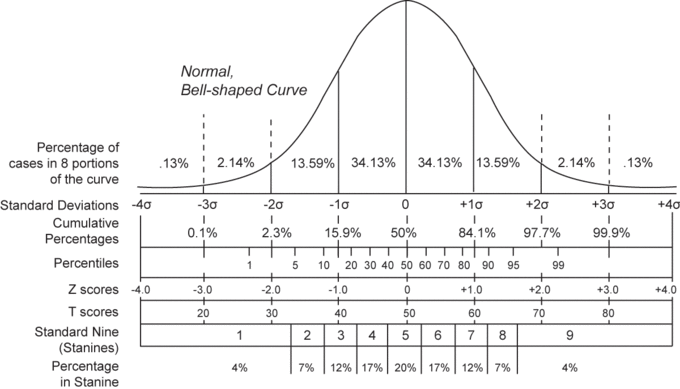Normal Distribution and Scales: Compares the various grading methods in a normal distribution. Includes: standard deviations, cumulative percentages, percentile equivalents, $\text{Z}$-scores, $\text{T}$-scores, and standard nine.